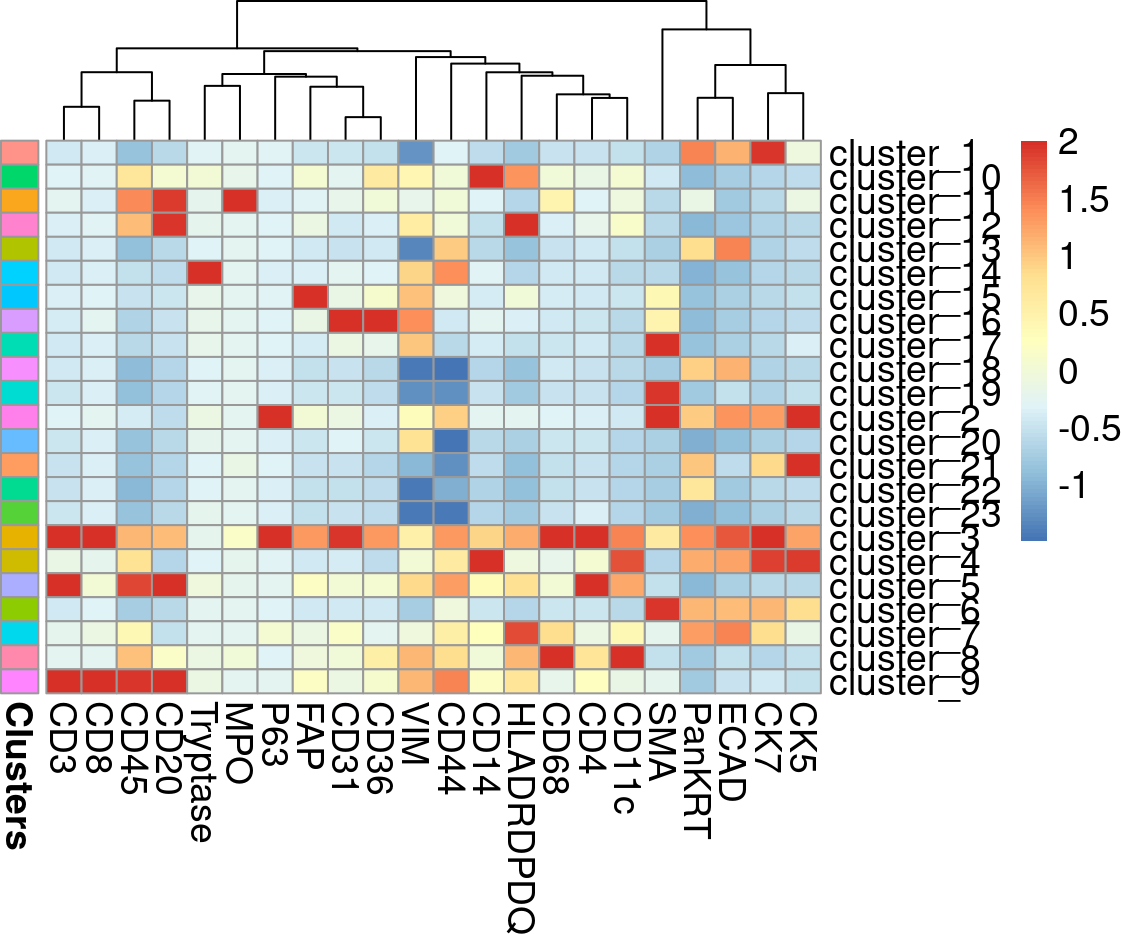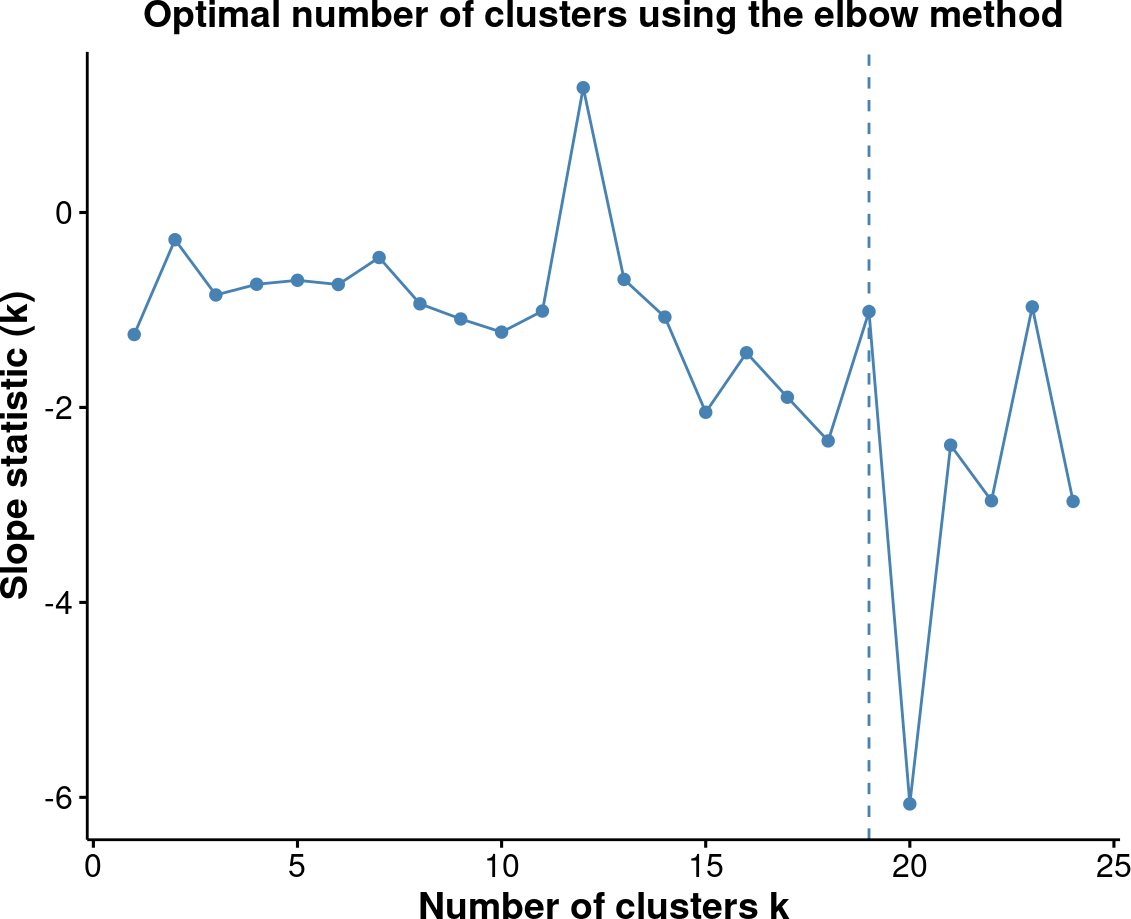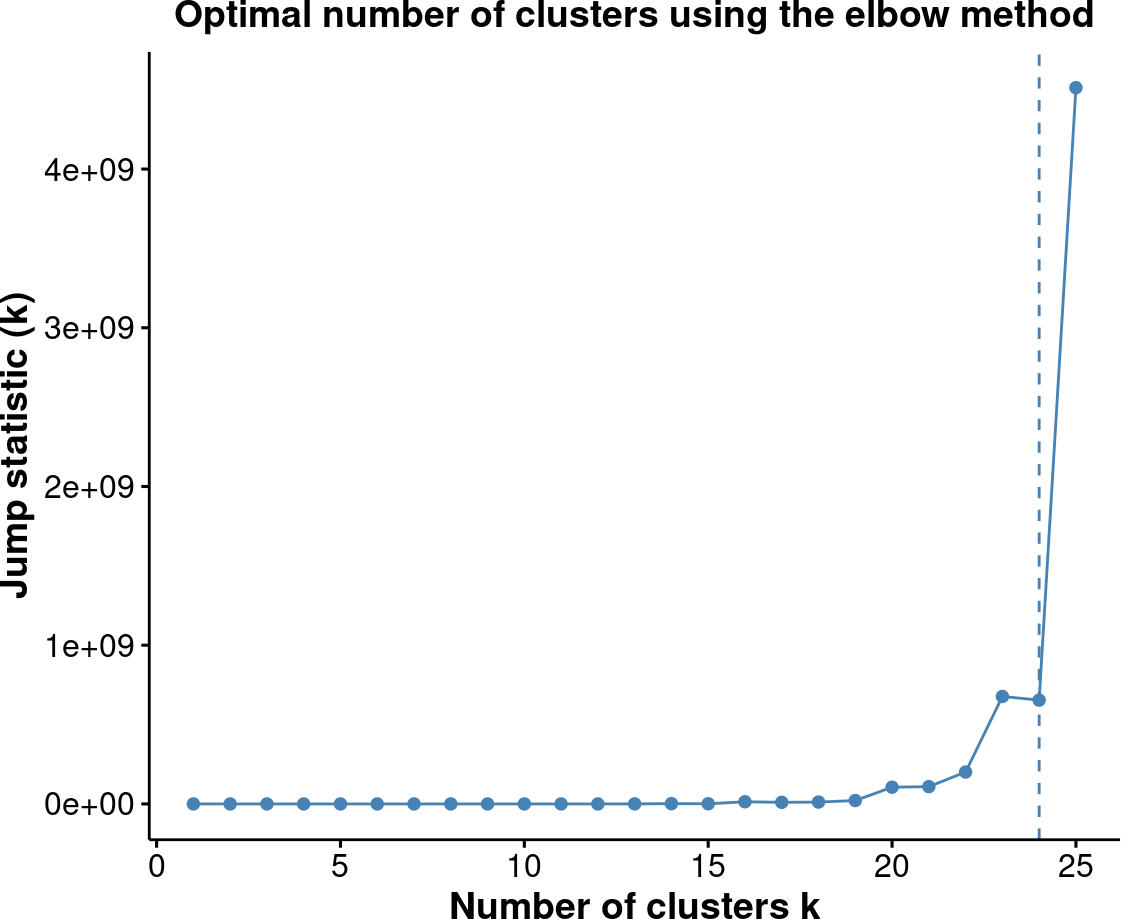# 1 Installation

``````if (!require("BiocManager"))
install.packages("BiocManager")
BiocManager::install("FuseSOM")``````

# 2 Introduction

A correlation based multiview self organizing map for the characterization of cell types (`FuseSOM`) is a tool for unsupervised clustering. `FuseSOM` is robust and achieves high accuracy by combining a `Self Organizing Map` architecture and a `Multiview` integration of correlation based metrics to cluster highly multiplexed in situ imaging cytometry assays. The `FuseSOM` pipeline has been streamlined and accepts currently used data structures including `SingleCellExperiment` and `SpatialExperiment` objects as well as `DataFrames`.

# 3 Disclaimer

This is purely a tool generated for clustering and as such it does not provide any means for QC and feature selection. It is advisable that the user first use other tools for quality control and feature selection before running `FuseSOM`.

# 4 Getting Started

## 4.1`FuseSOM` Matrix Input

If you have a matrix containing expression data that was QCed and normalised by some other tool, the next step is to run the `FuseSOM` algorithm.This can be done by calling the `runFuseSOM()` function which takes in the matrix of interest where the columns are markers and the rows are observations, the makers of interest (if this is not provided, it is assumed that all columns are markers), and the number of clusters.

``````# load FuseSOM
library(FuseSOM)``````

Next we will load in the `Risom et al` dataset and run it through the FuseSOM pipeline. This dataset profiles the spatial landscape of ductal carcinoma in situ (DCIS), which is a pre-invasive lesion that is thought to be a precursor to invasive breast cancer (IBC). The key conclusion of this manuscript (amongst others) is that spatial information about cells can be used to predict disease progression in patients.We will also be using the markers used in the original study.

``````# load in the data
data("risom_dat")

# define the markers of interest
'Tryptase','MPO','CD20','CD3','CD8','CD4','CD14','CD68','FAP',

# we will be using the manual_gating_phenotype as the true cell type to gauge
# performance
names(risom_dat)[names(risom_dat) == 'manual_gating_phenotype'] <- 'CellType'``````

Now that we have loaded the data and define the markers of interest. We can run the `FuseSOM` algorithm. We have provided a function `runFuseSOM` that runs the pipeline from top to bottom and returns the cluster labels as well as the `Self Organizing Map` model.

``````risomRes <- runFuseSOM(data = risom_dat, markers = risomMarkers,
numClusters = 23)``````
``## You have provided a dataset of class data.frame``
``## Everything looks good. Now running the FuseSOM algorithm``
``## Now Generating the Self Organizing Map Grid``
``## Optimal Grid Size is: 8``
``## Now Running the Self Organizing Map Model``
``## Now Clustering the Prototypes``
``## Loading required namespace: fastcluster``
``## Now Mapping Clusters to the Original Data``
``## The Prototypes have been Clustered and Mapped Successfully``
``## The FuseSOM algorithm has completed successfully``

Lets look at the distribution of the clusters.

``````# get the distribution of the clusters
table(risomRes\$clusters)/sum(table(risomRes\$clusters))``````
``````##
##   cluster_1  cluster_10  cluster_11  cluster_12  cluster_13  cluster_14
## 0.323602021 0.035968538 0.005439775 0.021443334 0.061100586 0.026596050
##  cluster_15  cluster_16  cluster_17  cluster_18  cluster_19   cluster_2
## 0.020582156 0.032624297 0.024931106 0.076128143 0.015802618 0.014927087
##  cluster_20  cluster_21  cluster_22  cluster_23   cluster_3   cluster_4
## 0.049962682 0.009185900 0.051771156 0.066913538 0.004923068 0.014108968
##   cluster_5   cluster_6   cluster_7   cluster_8   cluster_9
## 0.040776783 0.064444827 0.020854863 0.010032725 0.007879780``````

Looks like `cluster_1` has about \(32\%\) of the cells which is interesting. Next, lets generate a heatmap of the marker expression for each cluster.

``````risomHeat <- FuseSOM::markerHeatmap(data = risom_dat, markers = risomMarkers,
clusters = risomRes\$clusters, clusterMarkers = TRUE)``````## 4.2 Using `FuseSOM` to estimate the number of clusters

`FuseSOM` also provides functionality for estimating the number of clusters in a dataset using three classes of methods including:

1. Discriminant based method.
• A method developed in house based on discriminant based maximum clusterability projection pursuit
2. Distance based methods which includes:
• The Gap Statistic
• The Jump Statistic
• The Slope Statistic
• The Within Cluster Dissimilarity Statistic
• The Silhouette Statistic

We can estimate the number of clusters using the `estimateNumCluster`. Run `help(estimateNumCluster)` to see it’s complete functionality.

``````# lets estimate the number of clusters using all the methods
# original clustering has 23 clusters so we will set kseq from 2:25
# we pass it the som model generated in the previous step
risomKest <- estimateNumCluster(data = risomRes\$model, kSeq = 2:25,
method = c("Discriminant", "Distance"))``````
``## Now Computing the Number of Clusters using Discriminant Analysis``
``## Now Computing The Number Of Clusters Using Distance Analysis``

We can then use this result to determine the best number of clusters for this dataset based on the different metrics. The `FuseSOM` package provides a plotting function (`optiPlot`) which generates an elbow plot with the optimal value for the number of clusters for the distance based methods. See below

``````# what is the best number of clusters determined by the discriminant method?
# optimal number of clusters according to the discriminant method is 7
risomKest\$Discriminant ``````
``##  8``
``````# we can plot the results using the optiplot function
pSlope <- optiPlot(risomKest, method = 'slope')
pSlope````````````pJump <- optiPlot(risomKest, method = 'jump')
pJump````````````pWcd <- optiPlot(risomKest, method = 'wcd')
pWcd``````